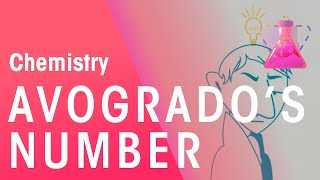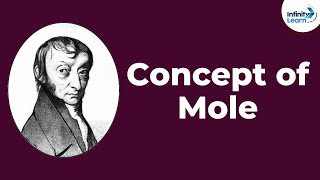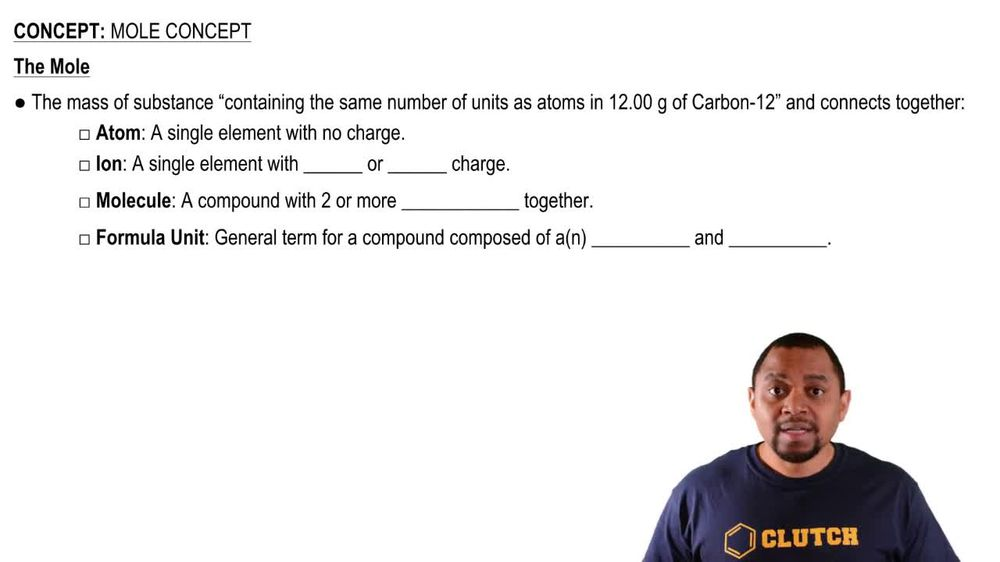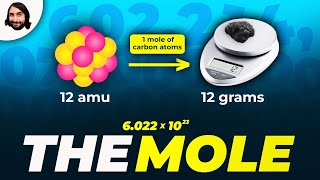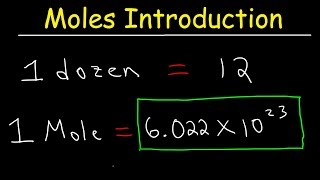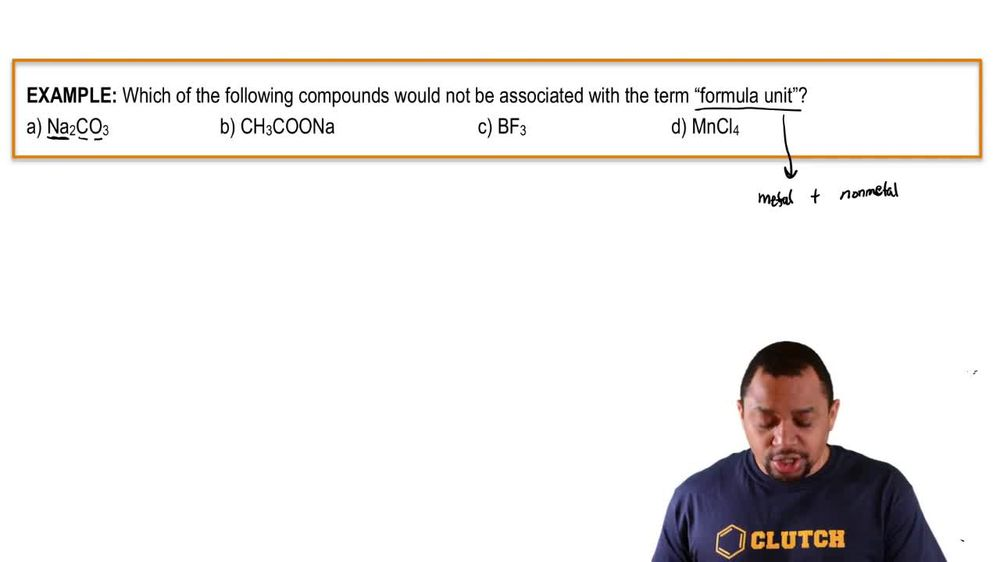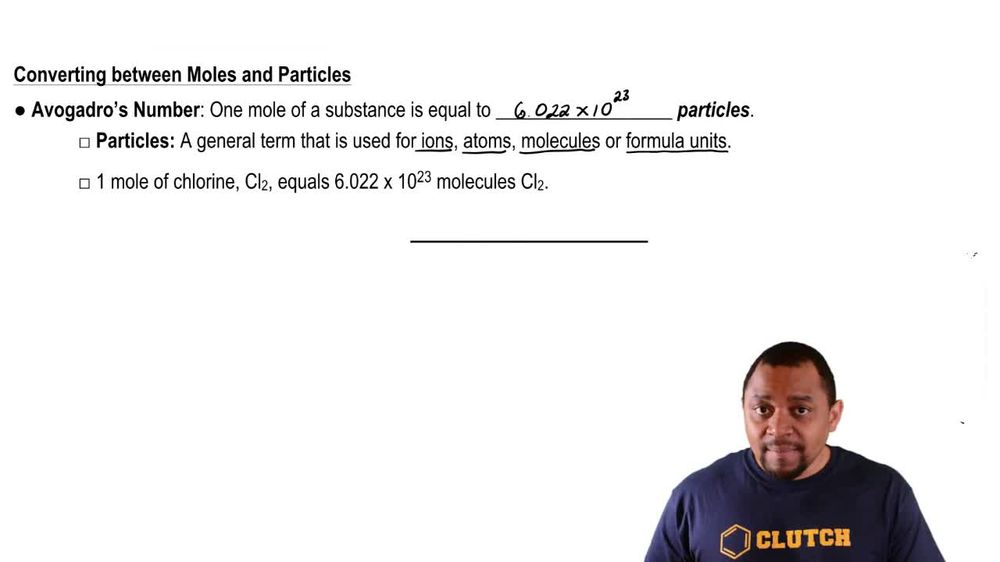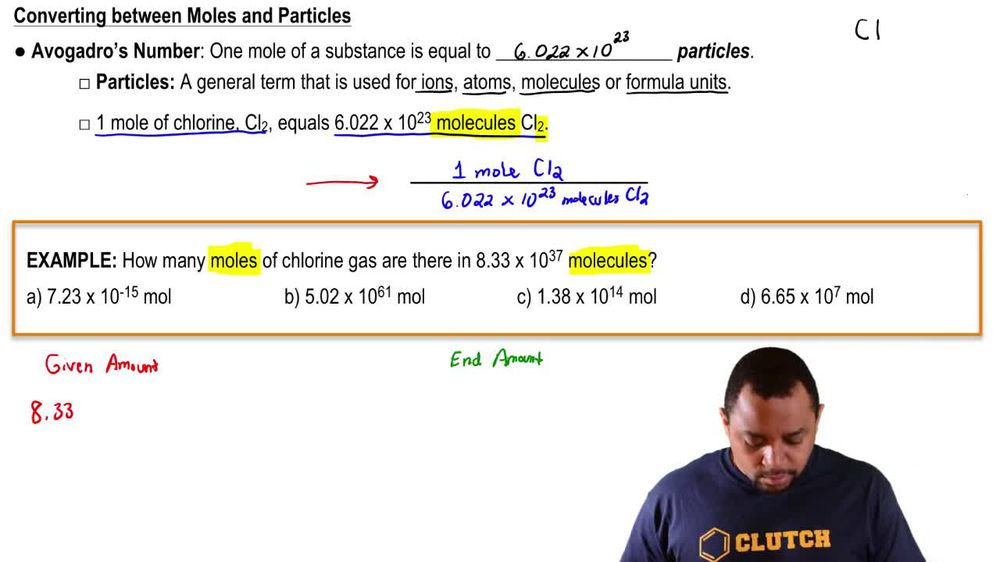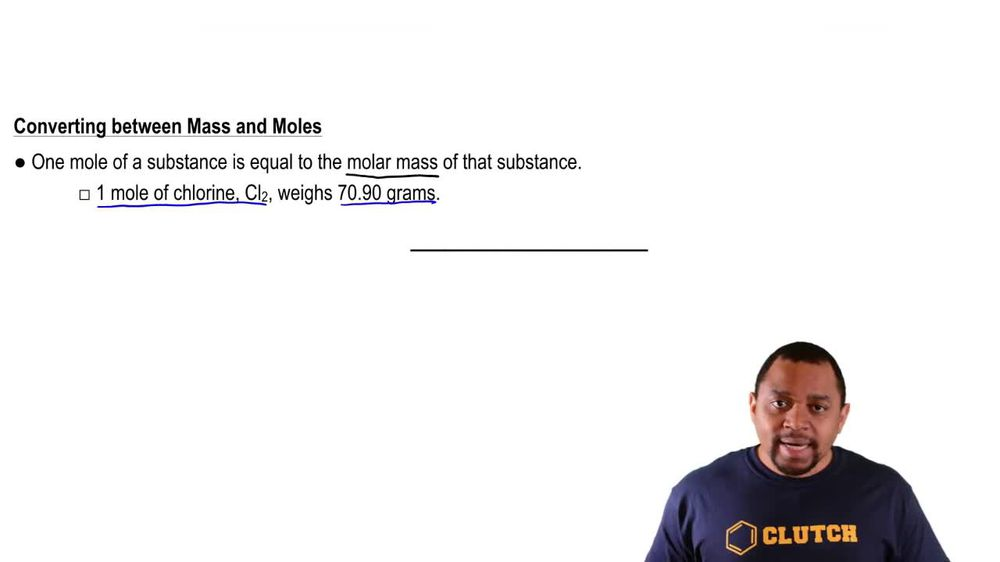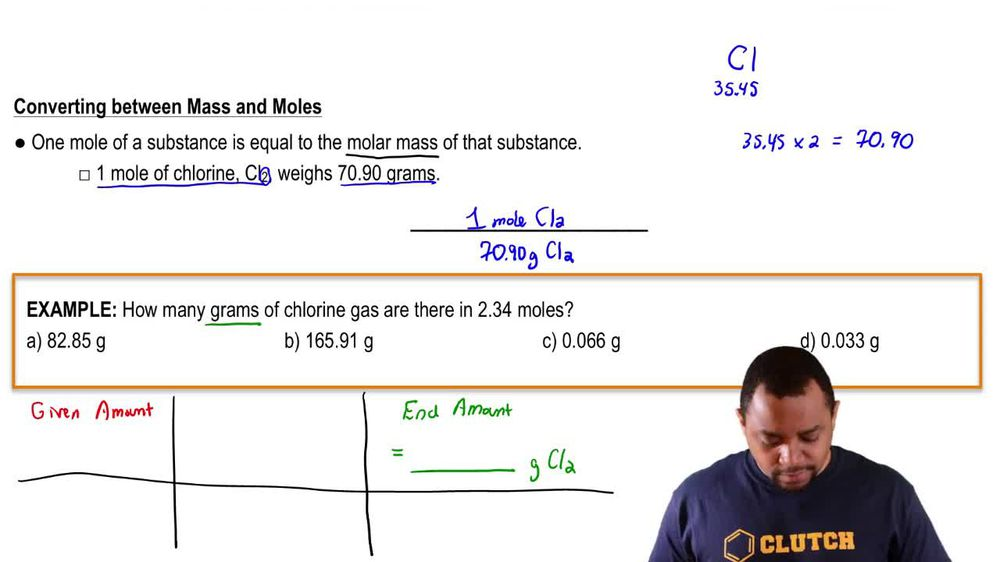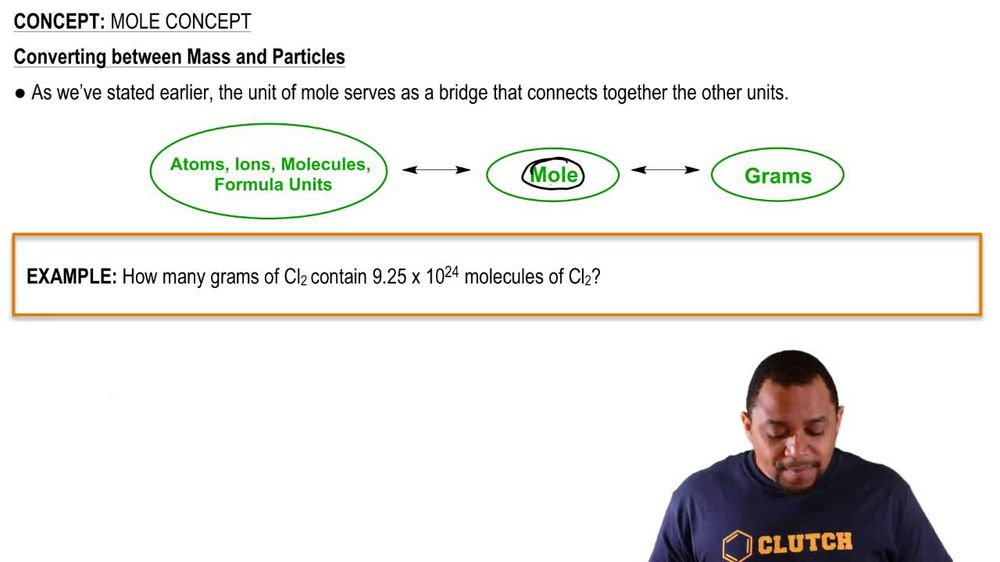Start typing, then use the up and down arrows to select an option from the list.
1. 2. Atoms & Elements2. Mole Concept
Problem

# Determine the number of moles of oxygen atoms in each sample. a. 4.88 mol H2O2 b. 2.15 mol N2O c. 0.0237 mol H2CO3 d. 24.1 mol CO2

Relevant Solution1m
Play a video:
Hi everyone today, we have a question asking us to determine the number of moles of phosphorus and three moles of calcium phosphate. So we're going to start out with our three moles of calcium phosphate and then we're going to multiply by two moles a phosphorus and it's two moles because of this too. We have right here outside of the parentheses, and that is going to be over one more of calcium far state. And so are moles of calcium phosphate, are going to cancel out, And that is going to equal six moles a phosphorus. And that is our final answer. Thank you for watching. Bye.